# C Type Casting

Type casting is a way to force an expression to be of a specific type by using a cast. Here is the general form of a cast:

`(type) expression;`

Here, type is any valid data type. Below is an example to cause the expression i/2 to evaluate to the type float.

`(float) i/2;`

Now let's take some example programs for complete understanding on type casting in C.

## C Type Casting Example

Here is an example, demonstrating type casting in C.

```/* C Type Casting - This C program illustrates
* the concept of type casting in C language
*/

#include<stdio.h>
#include<conio.h>
void main()
{
int i=3;
float fl;
clrscr();

fl = (float) i /2;
printf("The value is %f", fl);

getch();
}```

Here is the sample output of the above C program: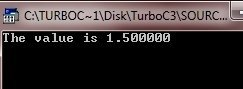Here is another program telling the advantage of casting an expression in a program.

```/* C Type Casting - This program tells the
* advantage of type casting in a program
*/

#include<stdio.h>
#include<conio.h>
void main()
{
int i=3, resi;
float resf;
clrscr();

resi = i/2;
resf = (float) i /2;

printf("If i is an int type variable having value 3. Then\n");
printf("i/2 = %d", resi);
printf("\n(float) i/2 = %f", resf);

getch();
}```

Here is the sample run of this C program: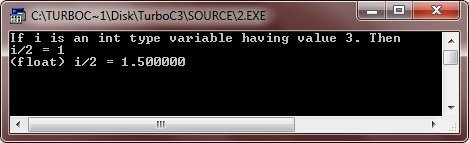Here is one more example program.

```#include<stdio.h>
#include<conio.h>
void main()
{
int i;
clrscr();

for(i=1; i<=10; i++)
{
printf("%d / 2 = %f\n", i, (float)i /2);
}

getch();
}```

Below is the sample run of this C program: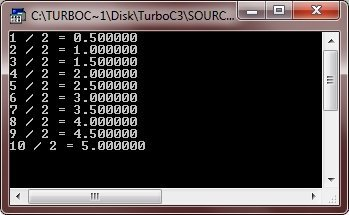Here is one more example. Just look at this for the complete understanding on type casting in C.

```#include<stdio.h>
#include<conio.h>
void main()
{
int mark, i, sum=0;
float avg;
clrscr();

printf("Enter marks obtained in 5 subjects: ");
for(i=0; i<5; i++)
{
scanf("%d", &mark[i]);
sum = sum + mark[i];
}

avg = (float) sum/5;

printf("Average Mark = %f", avg);

getch();
}```

Below is the sample run of the above C program: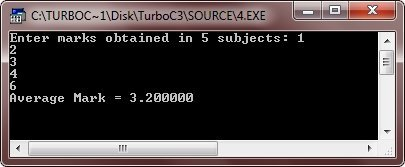## Integer Promotion in C

Integer promotion is a way by which values of integer type, smaller than int or unsigned int are converted either to an int or an unsigned int. Here is an example of adding a character in an int:

```#include<stdio.h>
#include<conio.h>
void main()
{
int i = 10, sum;
char ch = 'a';
clrscr();

sum = i + ch;    // ASCII values of a is 97
printf("If i is an int and ch is a character type.\n");
printf("And i has value 10 and ch has value 'a'.\n");
printf("The ASCII value of a is 97. Then\n\n");
printf("i + ch = %d", sum);

getch();

}```

On compile and executing the above code, it will produce the following result: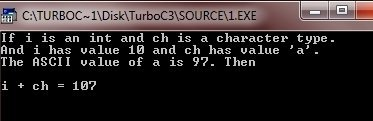As you can see from the above C program, the value of sum is 107 because the compiler is doing integer promotion here by converting the value of 'a' to ASCII which is 97 before performing the actual addition operation. Now let's take another example.

```#include<stdio.h>
#include<conio.h>
void main()
{
char ch1 = 'A';
char ch2 = 'a';
int i1, i2;
clrscr();

for(i1=65, i2=97; i2<=122; i1++, i2++, ch1++, ch2++)
{
printf("%c\t%c\t%c\t%c\n", i1, i2, ch1, ch2);
}

getch();

}```

Here is the sample run of the above C program. This program will print A-Z, a-z, A-Z, and again a-z in four vertical line: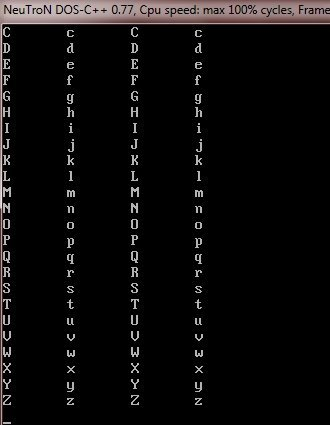You can also write the above C program like this one:

```#include<stdio.h>
#include<conio.h>
void main()
{
char ch1 = 'A';
char ch2 = 'a';
int i1=65, i2=97;
int l;
clrscr();

for(l=0; l<26; l++)
{
printf("%c\t%c\t%c\t%c\n", i1, i2, ch1, ch2);
i1++;
i2++;
ch1++;
ch2++;
}

getch();

}```

The output of this C program will be same as above one.

## Arithmetic Conversion in C

The usual arithmetic conversions in C language, are implicitly performed to cast their values to a common type. The C compiler first performs integer promotion, if the operands still have different types, then they are converted to the type that appears highest, given in this hierarchy: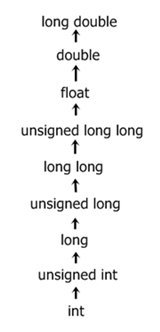Note - The usual arithmetic conversions in C language, can't performed for the assignment and logical operators (&& and ||).

Tools
Calculator